# Multiplication - 6th grade (11y) - math problems

#### Number of problems found: 196

• Expression 6Evaluate expression: -6-2(4-8)-9
• Quotient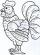Determine the quotient (q) and the remainder (r) from division numbers 100 and 8. Take the test of correctness.
• Number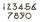What number I think? The third is 6 and half is 2 more than the its quarter.
• Division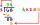Divide by the number 0.2 is the same as multiply by what number?
• Dinesh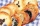Dinesh ate 3 cookies, each with mass 1.45 g. What was the total mass of the cookies that he ate?
• Brackets 2Add parenthesis to make true: 5-2×6-4+2=5
• Cube 3How many times will increase the volume of a cube if we double the length of its edge?
• The smallest numberWhat is the smallest number that can be divided by both 5 and 7
• LCMWhat is the least common multiple of 5, 50, 14?
• With bracket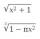Calculate (evaluate) simple mathematical expression with a negative numbers and a bracket: 13+15*5-2*(-6)
• Expressions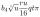Expression (3a-7).(3a+7) is identical with: ?
• Cube 2How many times will increase if the surface area of the cube if we triple length of its edge?
• SymbolsIf 2*3 = 60 ; 3*4 = 120 and 4*5 = 200, what is 2*5?
• Factors 2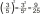A number has 3 identical factors. If 1 of them is 18. Find the number step by step
• Chess competition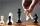4 chess players took part in the competition. How many tournaments have taken place if every chess player has fought everyone once?
• Lcm 2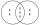Create the smallest possible number that is divisible by numbers 5,8,9,4,3
• Two thirdsFind two-thirds of the number equal to two-thirds of 99
• Aircraft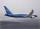If an airplane flies 776 km/h for 7.4 h, how far does it travel?
• Product of the sum and differenceCalculate the product of the sum and difference of numbers -7 and -2.
• Colors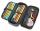Willie got birthday 6 colour pens in different colors. How many ways he can give them side by side in pencil?

Do you have an interesting mathematical word problem that you can't solve it? Submit a math problem, and we can try to solve it.

We will send a solution to your e-mail address. Solved examples are also published here. Please enter the e-mail correctly and check whether you don't have a full mailbox.

Please do not submit problems from current active competitions such as Mathematical Olympiad, correspondence seminars etc...